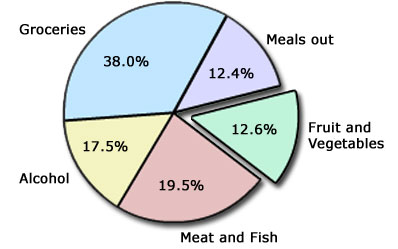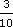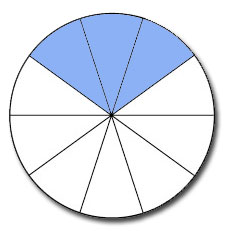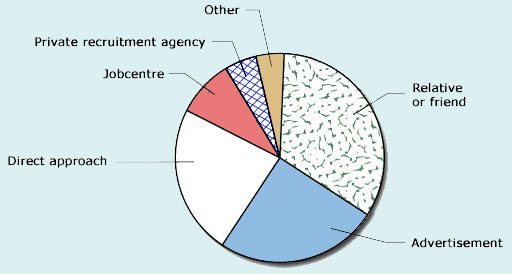Diagrams, charts and graphs

Start this free course now. Just create an account and sign in. Enrol and complete the course for a free statement of participation or digital badge if available.

Free course

# 2.3 Pie charts

Pie charts are representations that make it easy to compare proportions: in particular, they allow quick identification of very large proportions and very small proportions. They are generally based on large sets of data.

The pie chart below summarises the average weekly expenditure by a sample of families on food and drink. The whole circle represents 100% of the expenditure. The circle is then divided into ‘segments’, and the area of each segment represents a fraction or percentage of the total expenditure. For instance, groceries account for 38.0% of the total expenditure. The area of this slice is 38/100 of the total area.Pie charts can be constructed by dividing a circle into equal slices and then shading in the appropriate fractions. For example, the circle below is divided into 10 equal slices. The shaded area isor 30% of the total.Often, pie charts do not have the actual percentages or fractions marked on them, so it is difficult to glean any precise information. However, the percentages and fractions can be estimated.

## Example 5

This pie chart shows how a sample of people first heard about their present jobs. Interpret the pie chart by estimating the percentage of people in each category.The percentages can be estimated by eye. Thus the largest proportion (aboutof the circle or 33%) heard about their jobs from a relative or a friend. Aboutor 25% took the initiative from an advertisement, and a slightly smaller proportion than that, perhaps 22%, followed a direct approach. The three smaller slices are more difficult to estimate: those hearing about their jobs through a Jobcentre correspond to about 10%, while the slices for the private recruitment agency and ‘other’ are each perhaps half of that, and so individually correspond to about 5% of the total.

To check these estimates, add the percentages. The total should be close to 100%:

33 + 25 + 22 + 10 + 5 + 5 = 100%

Pie charts can also provide a good visual comparison of proportions arising from different sets of similar data. The pie charts below summarise the methods used by people to consult their doctors. Here the actual percentages are quoted on the slices of the pies. The pie chart on the left presents information relating to people of all ages; the pie chart on the right presents information relating to elderly people. It can be seen that in the population as a whole, most people (72.1%) consult their doctors at the surgery; by contrast, most elderly people (62.3%) are likely to be visited by their doctors at home.

## Example 6

In one year a mathematics tutor found that four of her students gained distinctions, six gained Grade 2 passes, one a Grade 3 pass, one a Grade 4 pass and the other four students withdrew during the course. Draw a pie chart to represent these data.

First, calculate how many students there were altogether:

4 + 6 + 1 + 1 + 4 = 16 students.

Divide the pie into 16 equal slices and mark the correct number of slices for each category of students. The slices should then be labelled appropriately.

MU120_4M5